# Initialization modules

Initialization modules allow to initialize the state variables using data previously computed by external software. The number of initialization module records is specified in analysis record using ninitmodules parameter (see the initial part of section 3). The general format is the following:
 *EntType initfile #(string)

The file name following the keyword initfile'' specifies the path to the file that contains the initialization data and should be given without quotes.

Currently, the only supported initialization module is

• Gauss point initialization module

 GPInitModule initfile #(string)

• Each Gauss point is represented by one line in the initialization file.
• The Gauss points should be given in a specific order, based on the element number and the Gauss point number, in agreement with the mesh specified in later sections.
• Each line referring to a Gauss point should contain the following data:

 elnum #(in) gpnum #(in) coords #(ra) ng #(in) var_1_id #(in) values_1 #(ra) ... var_ng_id #(in) values_ng #(ra)

• elnum is the element number
• gpnum is the Gauss point number
• coords are the coordinates of the Gauss point
• ng is the number of groups of variables that will follow
• var_1_id is the identification number of variable group number 1 (according to the definitions in internalstatetype.h)
• values_1 are the values of variables in group number 1
• var_ng_id is the identification number of variable group number ng
• values_ng are the values of variables in group number ng
• Example:
37 4 3 0.02 0.04 0.05 3 52 1 0.23 62 1 0.049 1 6 0 -2.08e+07 0 0 0 0'' means that Gauss point number 4 of element number 37 has coordinates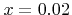,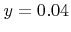and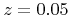and the initial values are specified for 3 groups of variables;
the first group (variable ID 52) is of type IST_DamageScalar (see internalstatetype.h) and contains 1 variable (since it is a scalar) with value 0.23;
the second group (ID 62) is of type IST_CumPlasticStrain and contains 1 variable with value 0.049;
the third group is of type IST_StressTensor and contains 6 variables (stress components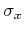,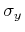, etc.) with values 0, -2.08e+07, 0, 0, 0, 0

Borek Patzak
2018-01-02